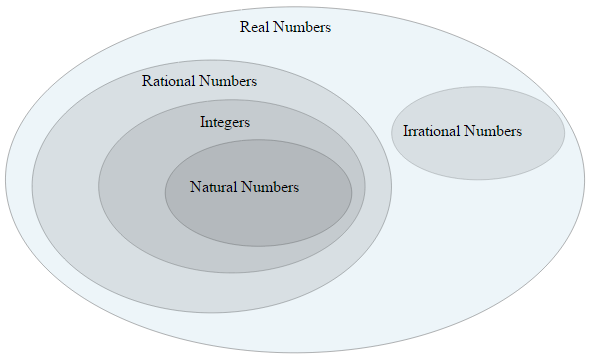# Classify this real number!

Calculus Level 4$\large \displaystyle \sum_{n=1}^\infty \dfrac {1}{2^{n^2}}$

In mathematics, a real number is a value that represents a quantity along a continuous line. The real numbers include all the rational numbers, irrational numbers. And some irrational numbers are transcendental.

What kind of number is the expression above?

×